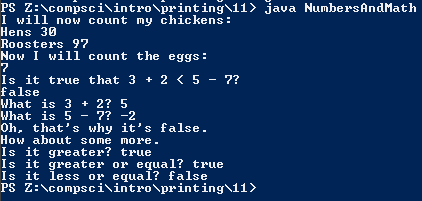# Assignment #11

## Code

```    /// Gian Adoremos
/// Period: 7
/// Program Name: Eleventh Program
/// File Name: NumbersAndMath.java
/// Date Finished: 9/11/2015

public class NumbersAndMath
{
public static void main( String[] args )
{
// This will print out "I will now count my chickens:"
System.out.println( "I will now count my chickens:" );

// This will print out the number of hens.
System.out.println( "Hens " + ( 25 + 30 / 6 ) );
// This will print out the number of roosters
System.out.println( "Roosters " + ( 100 - 25 * 3 % 4 ) );

// This will print out the text
System.out.println( "Now I will count the eggs:" );

// This will solve the equation
System.out.println( 3 + 2 + 1 - 5 + 4 % 2 - 1 / 4 + 6 );

// This will print out the text and solve the equation.
System.out.println( "Is it true that 3 + 2 < 5 - 7?" );

// This will solve the equation.
System.out.println( 3 + 2 < 5 - 7 );

// This will print the text and solve the equation.
System.out.println( "What is 3 + 2? " + ( 3 + 2 ) );
// This will print out the text and answer the equation.
System.out.println( "What is 5 - 7? " + ( 5 - 7 ) );

// This will print out the text.
System.out.println( "Oh, that's why it's false." );

//This will print out the text.
System.out.println( "How about some more." );

// This will print the text and solve the equation.
System.out.println( "Is it greater? " + ( 5 > -2 ) );
// This will print the text and solve the equation.
System.out.println( "Is it greater or equal? " + ( 5 >= -2 ) );
// This will print the text and solve the equation.
System.out.println( "Is it less or equal? " + ( 5 <= -2 ) );

}
}
```

### Picture of the output